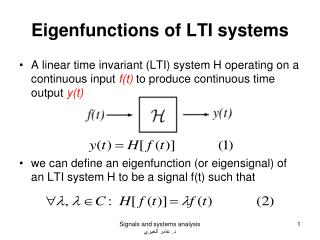Download PresentationEigenfunctions of LTI systems

# Eigenfunctions of LTI systems - PowerPoint PPT Presentation

Eigenfunctions of LTI systems. A linear time invariant (LTI) system H operating on a continuous input f(t) to produce continuous time output y(t) we can define an eigenfunction (or eigensignal) of an LTI system H to be a signal f(t) such that. Eigenfunctions of LTI systems.I am the owner, or an agent authorized to act on behalf of the owner, of the copyrighted work described.
Download Presentation## Eigenfunctions of LTI systems

Download Policy: Content on the Website is provided to you AS IS for your information and personal use and may not be sold / licensed / shared on other websites without getting consent from its author.While downloading, if for some reason you are not able to download a presentation, the publisher may have deleted the file from their server.

- - - - - - - - - - - - - - - - - - - - - - - - - - E N D - - - - - - - - - - - - - - - - - - - - - - - - - -
Presentation Transcript
1. Eigenfunctions of LTI systems • A linear time invariant (LTI) system H operating on a continuous input f(t) to produce continuous time output y(t) • we can define an eigenfunction (or eigensignal) of an LTI system H to be a signal f(t) such that Signals and systems analysis د. عامر الخيري

2. Eigenfunctions of LTI systems • Eigenfunctions are the simplest possible signals for H to operate on: to calculate the output, we simply multiply the input by a complex number. • The class of LTI systems has a set of eigenfunctions in common: the complex exponentials est, s  C, are eigenfunctions for all LTI systems. • Note: While {s, sC: est} are always eigenfunctions of an LTI system, they are not necessarily the only eigenfunctions. Signals and systems analysis د. عامر الخيري

3. Eigenfunctions of LTI systems • Proof of equation (3): Signals and systems analysis د. عامر الخيري

4. Eigenfunctions of LTI systems • Since the expression on the right hand side does not depend on t, it is a constant, s. Therefore, • The eigenvalue s is a complex number that depends on the exponent s and, of course, the system H. To make these dependencies explicit, we will use the notation H(s) s. Signals and systems analysis د. عامر الخيري

5. Eigenfunctions of LTI systems • Since the action of an LTI operator on its eigenfunctions est is easy to calculate and interpret, it is convenient to represent an arbitrary signal f(t) as a linear combination of complex exponentials. The Fourier series gives us this representation for periodic continuous time signals, while the (slightly more complicated) Fourier transform lets us expand arbitrary continuous time signals. Signals and systems analysis د. عامر الخيري